Courses

# Test: Single Correct MCQs: Functions | JEE Advanced

## 33 Questions MCQ Test Mathematics For JEE | Test: Single Correct MCQs: Functions | JEE Advanced

Description
This mock test of Test: Single Correct MCQs: Functions | JEE Advanced for JEE helps you for every JEE entrance exam. This contains 33 Multiple Choice Questions for JEE Test: Single Correct MCQs: Functions | JEE Advanced (mcq) to study with solutions a complete question bank. The solved questions answers in this Test: Single Correct MCQs: Functions | JEE Advanced quiz give you a good mix of easy questions and tough questions. JEE students definitely take this Test: Single Correct MCQs: Functions | JEE Advanced exercise for a better result in the exam. You can find other Test: Single Correct MCQs: Functions | JEE Advanced extra questions, long questions & short questions for JEE on EduRev as well by searching above.
QUESTION: 1

### Let R be the set of real numbers. If f : R → R is a function defined by f (x) = x2, then f is :

Solution:

f (x) = x2 is many one as f (1) = f (–1) = 1 Also f is into as – ve real number have no pre-image.
∴ F is neither injective nor surjective.

QUESTION: 2

### The entire graphs of the equation y = x2 + kx – x + 9 is strictly above the x-axis if and only if

Solution: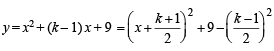For entire graph to be above x-axis, we should have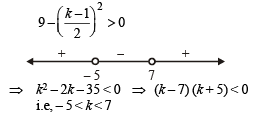QUESTION: 3

### Let f(x) = | x – 1 |. Then

Solution: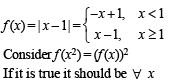∴ Put x = 2
LHS = f (22) = |4 – 1| = 3 and RHS = ( f (2))2 = 1
∴ (a) is not correct
Consider f (x + y) = f (x) + f (y)
Put x = 2, y = 5 we get
f (7) = 6; f (2) + f (5) = 1 + 4 = 5
∴ (b) is not correct
Consider f (| x |) = | f (x) |
Put x = – 5 then f (| –5 |) = f (5) = 4
| f (– 5) | = | – 5 – 1| = 6
∴ (c) is not correct.
Hence (d) is the correct alternative.

QUESTION: 4

If x satisfies |x - 1| + |x - 2| + |x - 3| > 6 , then

Solution: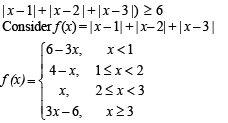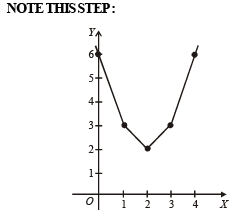Graph of f (x) shows f (x) > 6 for x < 0 or x > 4

QUESTION: 5

If f ( x) = cos(ln x), then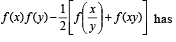the value

Solution: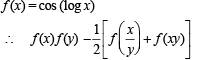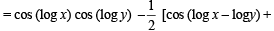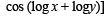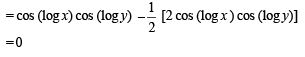QUESTION: 6

The domain of definition of the function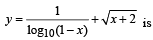Solution: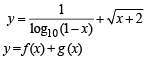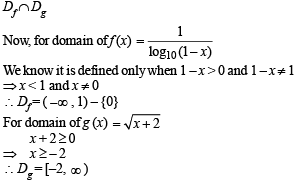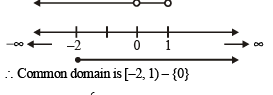QUESTION: 7

Which of the following functions is periodic?

Solution: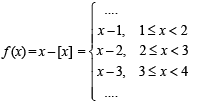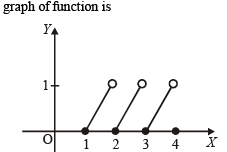Clearly it is a periodic function with period 1.
∴ (a) is the correct alternative.

QUESTION: 8

Let f(x) = sin x and g(x) = ln | x |. If th e ran ges of th e composition functions fog and gof are R1 and Rrespectively, th en

Solution: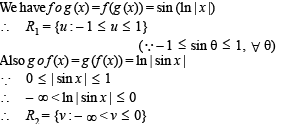QUESTION: 9

Let f ( x) = ( x + 1)2 - 1,x > -1 . Th en th e set {x : f (x) = f -1 (x)} is

Solution: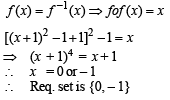QUESTION: 10

Th e fun ction f(x) = |px – q| + r | x |, x ∈ (-∞,∞) where p > 0, q > 0, r > 0  assumes its minimum value only on one point if

Solution: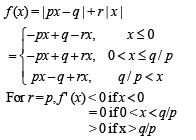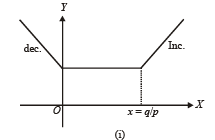From graph (i) infinite many points for min value of f (x)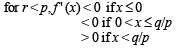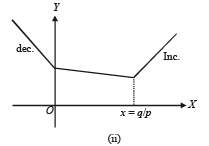From graph (ii) only pt. of min of f (x) at x = q/p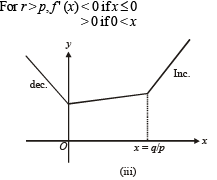From graph (iii) only one pt. of min of f (x) at x = 0

QUESTION: 11

Let f(x) be defined for all x > 0 and be continuous. Let f(x)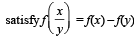for all x, y and f(e) = 1. Then

Solution:

f (x) is continuous and defined for all x > 0 and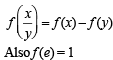⇒ Clearly f (x) = ln x which satisfies all these properties
∴ f (x) = ℓnx

QUESTION: 12

If the function f: [1, ∞) → [1, ∞) is defined by f(x) = 2x (x-1), then f–1 (x) is

Solution: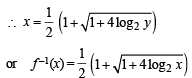QUESTION: 13

Let f : R → R be any function. Define g : R → R by g(x) = |f(x)| for all x.  Then g is

Solution:

Let h (x) = |x| then g (x) = |f (x)| = h (f (x))
Since composition of two continuous functions is continuous, therefore g is continuous if f is continuous.

QUESTION: 14

The domain of definition of the function f(x) given by the equation 2x + 2y = 2 is

Solution: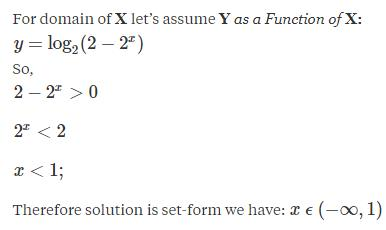QUESTION: 15

Let g(x) = 1 + x - [x] and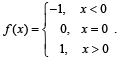Then for allx, f(g(x)) is equal to

Solution: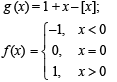For integral values of x; g (x) = 1
For x < 0; (but not integral value) x – [x] > 0 ⇒ g (x) > 1
For x > 0;(but not integral value) x – [x] > 0 ⇒ g (x) >1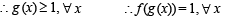QUESTION: 16

If f:[1, ∞) → [2, ∞) is given by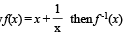equals

Solution: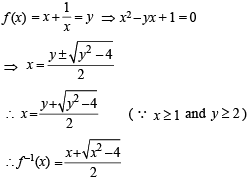QUESTION: 17

The domain of definition of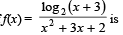Solution: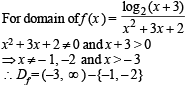QUESTION: 18

Let E = {1, 2, 3, 4} and F = {1, 2}. Then the number of onto functions from E to F is

Solution:

From E to F we can define, in all, 2 × 2 × 2 × 2 = 16 functions (2 options for each element of E) out of which 2 are into, when all the elements  of E either map to 1 or to 2.
∴ No. of onto functions = 16  – 2 = 14

QUESTION: 19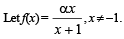Then, for what value of a is f (f(x)) = x ?

Solution: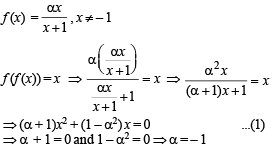QUESTION: 20

Suppose f(x) = (x + 1)2 for x > -1. If g(x) is the function whose graph is the reflection of the graph of f (x) with respect to the line y = x, then g(x) equals

Solution:

Given that f (x) = (x + 1)2, x > – 1
Now if g (x) is the reflection of f (x) in the line y = x then it can be obtained by interchanging x and y in f (x) i.e., y = (x + 1)2 changes to x = (y + 1)2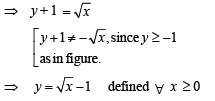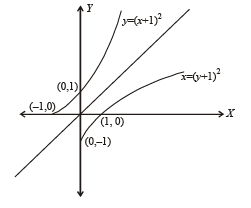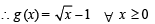QUESTION: 21

Let f unction f : R → R be defined by f(x) = 2x + sin x for x ∈ R , then f is

Solution: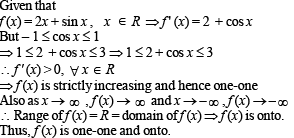QUESTION: 22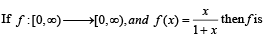Solution: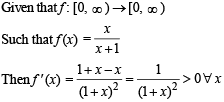∴ f is an increasing function ⇒ f is one-one.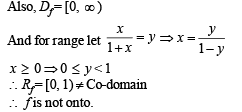QUESTION: 23

Domain of definition of the function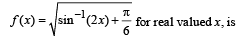Solution: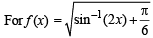to be defined and real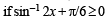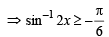...(1) But we know that – π/2 < sin–1 2x < π/2 ...(2) Combining (1) and (2), we get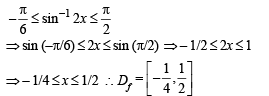QUESTION: 24

Range of the function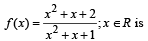Solution:

We have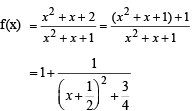We can see here that as x → ∞ , f (x) → 1 which is the min value of f (x). i.e. fmin = 1. Also f (x) is max when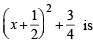min which is so when x  = – 1/2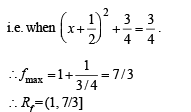QUESTION: 25

If f (x) = x2 + 2bx + 2c2 and g (x) = - x2 -2cx+ b2 such that min f (x) > max g (x), then the relation between b and c, is

Solution: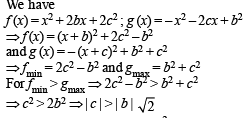QUESTION: 26

If f(x) = sin x + cos x, g (x) = x2 – 1, then g (f(x)) is invertible in the domain

Solution: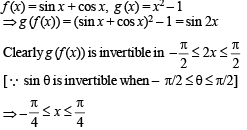QUESTION: 27

If the functions f(x) and g(x) are defined on R → R such that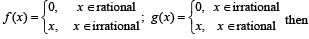Solution: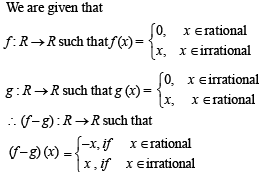Since f  –  g : R → R for any x there is only one value of (f (x) – g(x)) whether x is rational or irrational.
Moreover as x∈R, f (x) – g (x) also belongs to R.
Therefore, (f – g) is one-one onto.

QUESTION: 28

X an d Y are two sets and f : X → Y. If {f(c) = y; c ⊂ X, y ⊂ Y} and {f–1(d) = x; d ⊂ Y, x ⊂ X}, then the true statement is

Solution:

Given that X and Y are two sets and f : X → Y.
{f (c) = y; c ⊂ X, y ⊂ Y} and
{f –1(d) = x : d ⊂ Y, x ⊂ X }
The pictorial representation of given information is as shown: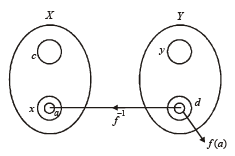Since f –1 (d) =  x ⇒ f (x) = d Now if a ⊂ x
⇒ f (a) ⊂ f (x) = d ⇒ f –1 [f (a)]  = a
∴ f –1 (f (a)) = a, a ⊂ x is the correct option.

QUESTION: 29

If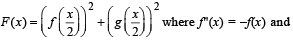g(x) = f '(x) and given that F(5) = 5, then F(10) is equal to

Solution: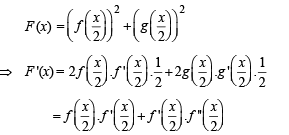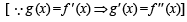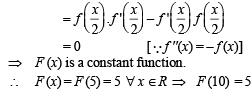QUESTION: 30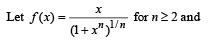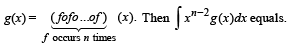Solution: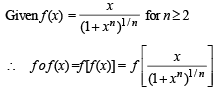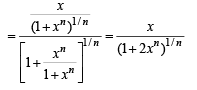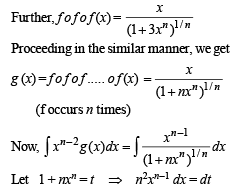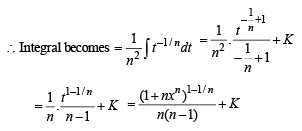QUESTION: 31

Let f, g and h be real-valued functions defined on the interval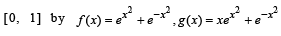and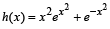. If a, b and c denote, respectively, the absolute maximum of f, g and h on [0, 1], then

Solution: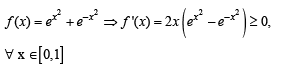∴ f (x) is an increasing function on [ 0,1]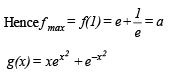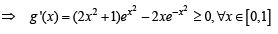∴ g (x) is an increasing function on [ 0,1]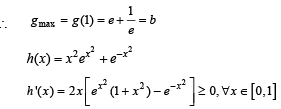∴ h (x) is an increasing function on [ 0,1]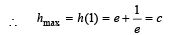Hence a = b = c.

QUESTION: 32

Let f (x) = x2 and g(x) = sin x for all x ∈ R. Then the set of all x satisfying (f o g o g o f) (x) = (g o g o f) (x), where (f o g) (x) = f (g(x)), is

Solution: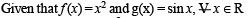Then (gof) (x) = sin x2
⇒ (gogof) (x) = sin (sin x2)
⇒ (fogogof) (x) = sin 2 (sin x2) As given that (fogogof) (x) = (gogof) (x)
⇒ sin2 (sin x2) = sin (sin x2)
⇒ sin (sin x2) = 0,1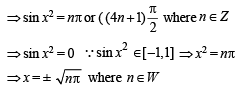QUESTION: 33

Th e function f : [0, 3] → [1, 29], defi n ed by f(x) = 2x3  – 15x2 + 36x + 1, is

Solution:

We have  f(x) = 2x3 – 15x2 + 36x + 1
⇒ f (x') = 6x2 – 30x + 36
=  6(x2 – 5x + 6)
=  6 (x – 2) (x – 3)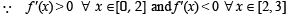∴ f(x) is in creasing on [0, 2] and decreasing on [2, 3]
∴ f(x) is many one on [0, 3]
Also f(0) = 1, f(2) = 29, f(3) = 28
∴ Global min = 1 and Global max = 29 i.e.,  Range of f = [1, 29] = codomain
∴ f is onto.